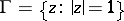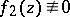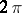# Luzin examples

(diff) ← Older revision | Latest revision (diff) | Newer revision → (diff)

in the theory of functions of a complex variable

Examples that characterize boundary uniqueness properties of analytic functions (see , ).

1) For any setof measure zero on the unit circle, N.N. Luzin constructed (1919, see ) a functionthat is regular, analytic and bounded in the unit discand is such thatdoes not have radial boundary values along each of the radii that end at points of.

A similar example of Luzin and I.I. Privalov (1925, see , ) differs only by insignificant changes.

2) Luzin also constructed (1925, see ) regular analytic functionsandinthat tend, respectively, to infinity and zero along all radii that end at points of some set of full measureon. This setis of the first Baire category (cf. Baire classes) on.

How to Cite This Entry:
Luzin examples. Encyclopedia of Mathematics. URL: http://encyclopediaofmath.org/index.php?title=Luzin_examples&oldid=18316
This article was adapted from an original article by E.D. Solomentsev (originator), which appeared in Encyclopedia of Mathematics - ISBN 1402006098. See original article